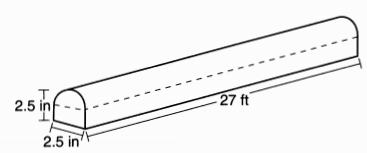mycollegehive
How much metal, to the nearest cubic inch, will the railing contain?
JMAP math geometry0A fabricator is hired to make a 27-foot-long solid metal railing for the stairs at the local library. The railing is modeled by the diagram below. The railing is 2.5 inches high and 2.5 inches wide and is comprised of a rectangular prism and a half-cylinder.How much metal, to the nearest cubic inch, will the railing contain?

(1) 151

(2) 795

(3) 1808

(4) 2025

55 viewsShareFollowUniversity of Benin Nigeria
11 June 2020University of Lagos Nigeria
30 August 20200The amount of metal the railing will contain is simply the volume of the railing.

Since the railing comprises of a rectangular prism and half a cylinder, the volume of the railing = volume of rectangular prism + half the volume of a cylinder.

Volume of a rectangular prism = L x H x W.

Where H is the height of the rectangular section of the railing, H is the height of the rectangular section of the railing and W is the width of the rectangular section of the railing.

The length of the rectangular section of the railing = 27 ft

To convert 27 ft to inch, multiply 27 by 12

Thus, the length of the rectangular section of the railing in inch = 27 x 12 = 324 inches.

From the diagram, the height of the rectangular section of the railing is half the entire height. That is, 2.5/2 = 1.25

Therefore, the volume of the rectangular section of the railing = 1.25 x 2.5 x 324

= 1012.5

Volume of a cylinder = πr2h. Where r is the radius of the cylindrical section and h is the height.

The height of the cylindrical section = 27 ft or 324 inches

Diameter of the cylindrical section = 2.5

radius = diameter/2 = 2.5/2 = 1.25

volume of the cylindrical section = π x (1.25)2 x 324

= 1590.43

Thus, half the volume = 1590.43/2

= 795.2

Therefore, the amount of metal the railing will contain = 1012.5 + 795.2

= 1808

Ans 3Share### Related Tags

JMAP

2 followers

143 questionsmath

8 followers

1015 questionsgeometry

1 followers

99 questions